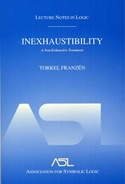# Lecture Notes in Logic 16

## Inexhaustibility: A Non-Exhaustive Treatment

Torkel FranzenYear: 2003
ISBN: 1-56881-174-8
300 pages. Hardcover.

Year: 2003
ISBN: 1-56881-175-6
300 pages. Paperback.

Gödel’s Incompleness Theorems are among the most significant results in the foundation of mathematics. These results have a positive consequence: any system of axioms for mathematics that we recognize as correct can be properly extended by adding as a new axiom a formal statement expressing that the original system is consistent. This suggests that our mathematical knowledge is inexhaustible, an essentially philosophical topic to which this book is devoted. Basic material in predicate logic, set theory and recursion theory is presented, leading to a proof of incompleteness theorems. The inexhaustibility of mathematical knowledge is treated based on the concept of transfinite progressions of theories as conceived by Turing and Feferman. All concepts and results necessary to understand the arguments are introduced as needed, making the presentation self-contained and thorough.

1. Introduction
2. Arithmetical Preliminaries
3. Primes and Proofs
4. The Language of Arithmetic
5. The Language of Analysis
6. Ordinals and Inductive Definitions
7. Formal Languages and the Definition of Truth
8. Logic and Theories
9. Peano Artithmetic and Computability
10. Elementary and Classical Analysis
11. The Recursion Theorem and Ordinal Notations
12. The Incompleteness Theorems
13. Iterated Consistency
14. Iterated Reflection
15. Iterated Iteration and Inexhaustibility